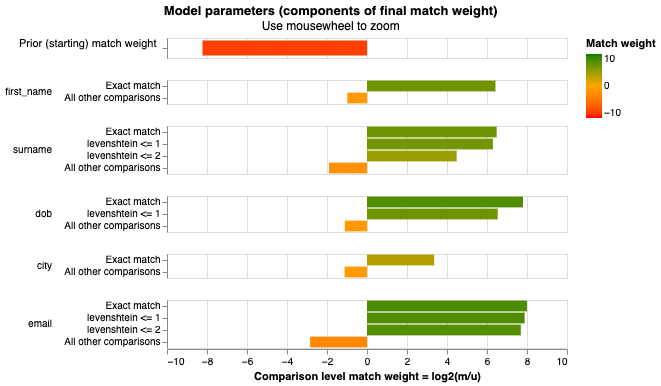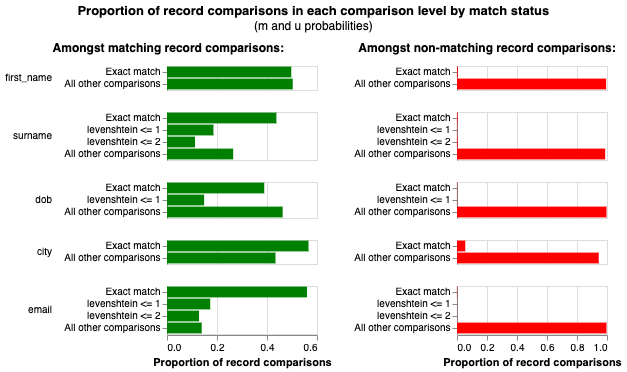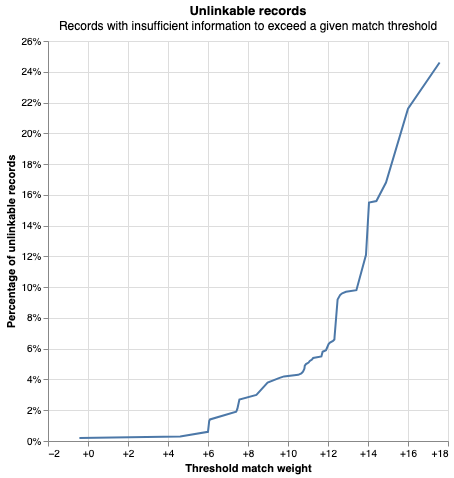# Specifying and estimating a linkage model¶

In the last tutorial we looked at how we can use blocking rules to generate pairwise record comparisons.

Now it's time to estimate a probabilistic linkage model to score each of these comparisons. The resultant match score is a prediction of whether the two records represent the same entity (e.g. are the same person).

The purpose of estimating the model is to learn the relative importance of different parts of your data for the purpose of data linking.

For example, a match on date of birth is a much stronger indicator that two records refer to the same entity than a match on gender. A mismatch on gender may be a stronger indicate against two records referring than a mismatch on name, since names are more likely to be entered differently.

The relative importance of different information is captured in the (partial) 'match weights', which can be learned from your data. These match weights are then added up to compute the overall match score.

The match weights are are derived from the m and u parameters of the underlying Fellegi Sunter model. Splink uses various statistical routines to estimate these parameters. Further details of the underlying theory can be found here, which will help you understand this part of the tutorial.

# Begin by reading in the tutorial data again
import pandas as pd
import altair as alt
alt.renderers.enable("mimetype")


To build a linkage model, the user defines the partial match weights that splink needs to estimate. This is done by defining how the information in the input records should be compared.

To be concrete, here is an example comparison:

first_name_l first_name_r surname_l surname_r dob_l dob_r city_l city_r email_l email_r
Robert Rob Allen Allen 1971-05-24 1971-06-24 nan London roberta25@smith.net roberta25@smith.net

What functions should we use to assess the similarity of Rob vs. Robert in the the first_name field?

Should similarity in the dob field be computed in the same way, or a different way?

Your job as the developer of a linkage model is to decide what comparisons are most appropriate for the types of data you have.

Splink can then estimate how much weight to place on a fuzzy match of Rob vs. Robert, relative to an exact match on Robert, or a non-match.

Defining these scenarios is done using Comparisons.

### Comparisons¶

The concept of a Comparison has a specific definition within Splink: it defines how data from one or more input columns is compared, using SQL expressions to assess similarity.

For example, one Comparison may represent how similarity is assessed for a person's date of birth.

Another Comparison may represent the comparison of a person's name or location.

A model is composed of many Comparisons, which between them assess the similarity of all of the columns being used for data linking.

Each Comparison contains two or more ComparisonLevels which define n discrete gradations of similarity between the input columns within the Comparison.

As such ComparisonLevelsare nested within Comparisons as follows:

Data Linking Model
├─-- Comparison: Date of birth
│    ├─-- ComparisonLevel: Exact match
│    ├─-- ComparisonLevel: One character difference
│    ├─-- ComparisonLevel: All other
├─-- Comparison: Surname
│    ├─-- ComparisonLevel: Exact match on surname
│    ├─-- ComparisonLevel: All other
│    etc.


Our example data would therefore result in the following comparisons, for dob and surname:

dob_l dob_r comparison_level interpretation
1971-05-24 1971-05-24 Exact match great match
1971-05-24 1971-06-24 One character difference ok match
1971-05-24 2000-01-02 All other bad match
surname_l surname_r comparison_level interpretation
Rob Rob Exact match great match
Rob Jane All other bad match
Rob Robert All other bad match, this comparison has no notion of nicknames

We will now use these concepts to build a data linking model.

### Specifying the model using comparisons¶

Splink includes libraries of comparison functions to make it simple to get started:

Let's start by looking at a Comparison for first_name:

import splink.duckdb.duckdb_comparison_library as cl

first_name_comparison = cl.levenshtein_at_thresholds("first_name", 2)

Comparison 'Exact match vs. levenshtein at threshold 2 vs. anything else' of "first_name".
Similarity is assessed using the following ComparisonLevels:
- 'Null' with SQL rule: "first_name_l" IS NULL OR "first_name_r" IS NULL
- 'Exact match' with SQL rule: "first_name_l" = "first_name_r"
- 'levenshtein <= 2' with SQL rule: levenshtein("first_name_l", "first_name_r") <= 2
- 'All other comparisons' with SQL rule: ELSE




## Specifying the full settings dictionary¶

Comparisons are specified as part of the Splink settings, a Python dictionary which controls all of the configuration of a Splink model:

settings = {
"comparisons": [
cl.exact_match("first_name"),
cl.levenshtein_at_thresholds("surname"),
cl.levenshtein_at_thresholds("dob", 1),
cl.levenshtein_at_thresholds("email"),
],
"blocking_rules_to_generate_predictions": [
"l.first_name = r.first_name",
"l.surname = r.surname",
],
"retain_matching_columns": True,
"retain_intermediate_calculation_columns": True,
}



In words, this setting dictionary says:

• We are performing a dedupe_only (the other options are link_only, or link_and_dedupe, which may be used if there are multiple input datasets).
• When comparing records, we will use information from the first_name, surname, dob, city and email columns to compute a match score.
• The blocking_rules_to_generate_predictions states that we will only check for duplicates amongst records where either the first_name or surname is identical.
• We have enabled term frequency adjustments for the 'city' column, because some values (e.g. London) appear much more frequently than others.
• We have set retain_intermediate_calculation_columns and additional_columns_to_retain to True so that Splink outputs additional information that helps the user understand the calculations. If they were False, the computations would run faster.

## Estimate the parameters of the model¶

Now that we have specified our linkage model, we need to estimate the probability_two_random_records_match, u, and m parameters.

• The probability_two_random_records_match parameter is the probability that two records taken at random from your input data represent a match (typically a very small number).

• The u values are the proportion of records falling into each ComparisonLevel amongst truly non-matching records.

• The m values are the proportion of records falling into each ComparisonLevel amongst truly matching records

We can estimate these parameters using unlabeled data. If we have labels, then we can estimate them even more accurately.

### Estimation of probability_two_random_records_match¶

In some cases, the probability_two_random_records_match will be known. For example, if you are linking two tables of 10,000 records and expect a one-to-one match, then you should set this value to 1/10_000 in your settings instead of estimating it.

More generally, this parameter is unknown and needs to be estimated.

It can be estimated accurately enough for most purposes by combining a series of deterministic matching rules and a guess of the recall corresponding to those rules. For further details of the rationale behind this appraoch see here.

In this example, I guess that the following deterministic matching rules have a recall of about 70%:

deterministic_rules = [
"l.first_name = r.first_name and levenshtein(r.dob, l.dob) <= 1",
"l.surname = r.surname and levenshtein(r.dob, l.dob) <= 1",
"l.first_name = r.first_name and levenshtein(r.surname, l.surname) <= 2",
"l.email = r.email"
]


Probability two random records match is estimated to be  0.00333.
This means that amongst all possible pairwise record comparisons, one in 300.13 are expected to match.  With 499,500 total possible comparisons, we expect a total of around 1,664.29 matching pairs



### Estimation of u probabilities¶

Once we have the probability_two_random_records_match parameter, we can estimate the u probabilities.

We estimate u using the estimate_u_using_random_sampling method, which doesn't require any labels.

It works by sampling random pairs of records, since most of these pairs are going to be non-matches. Over these non-matches we compute the distribution of ComparisonLevels for each Comparison.

For instance, for gender, we would find that the the gender matches 50% of the time, and mismatches 50% of the time.

For dob on the other hand, we would find that the dob matches 1% of the time, has a "one character difference" 3% of the time, and everything else happens 96% of the time.

The larger the random sample, the more accurate the predictions. You control this using the target_rows parameter. For large datasets, we recommend using at least 10 million - but the higher the better and 1 billion is often appropriate for larger datasets.

linker.estimate_u_using_random_sampling(target_rows=1e6)

----- Estimating u probabilities using random sampling -----

Estimated u probabilities using random sampling

Your model is not yet fully trained. Missing estimates for:
- first_name (no m values are trained).
- surname (no m values are trained).
- dob (no m values are trained).
- city (no m values are trained).
- email (no m values are trained).



### Estimation of m probabilities¶

m is the trickiest of the parameters to estimate, because we have to have some idea of what the true matches are.

If we have labels, we can directly estimate it.

If we do not have labelled data, the m parameters can be estimated using an iterative maximum likelihood approach called Expectation Maximisation.

#### Estimating directly¶

If we have labels, we can estimate m directly using the estimate_m_from_label_column method of the linker.

For example, if the entity being matched is persons, and your input dataset(s) contain social security number, this could be used to estimate the m values for the model.

Note that this column does not need to be fully populated. A common case is where a unique identifier such as social security number is only partially populated.

For example (in this tutorial we don't have labels, so we're not actually going to use this):

linker.estimate_m_from_label_column("social_security_number")


#### Estimating with Expectation Maximisation¶

This algorithm estimates the m values by generating pairwise record comparisons, and using them to maximise a likelihood function.

Each estimation pass requires the user to configure an estimation blocking rule to reduce the number of record comparisons generated to a manageable level.

In our first estimation pass, we block on first_name and surname, meaning we will generate all record comparisons that have first_name and surname exactly equal.

Recall we are trying to estimate the m values of the model, i.e. proportion of records falling into each ComparisonLevel amongst truly matching records.

This means that, in this training session, we cannot estimate parameter estimates for the first_name or surname columns, since they will be equal for all the comparisons we do.

We can, however, estimate parameter estimates for all of the other columns. The output messages produced by Splink confirm this.

training_blocking_rule = "l.first_name = r.first_name and l.surname = r.surname"


----- Starting EM training session -----

Estimating the m probabilities of the model by blocking on:
l.first_name = r.first_name and l.surname = r.surname

Parameter estimates will be made for the following comparison(s):
- dob
- city
- email

Parameter estimates cannot be made for the following comparison(s) since they are used in the blocking rules:
- first_name
- surname

Iteration 1: Largest change in params was -0.514 in the m_probability of dob, level Exact match
Iteration 2: Largest change in params was 0.0474 in probability_two_random_records_match
Iteration 3: Largest change in params was 0.0212 in probability_two_random_records_match
Iteration 4: Largest change in params was 0.0113 in probability_two_random_records_match
Iteration 5: Largest change in params was 0.00694 in probability_two_random_records_match
Iteration 6: Largest change in params was 0.00463 in probability_two_random_records_match
Iteration 7: Largest change in params was 0.00328 in probability_two_random_records_match
Iteration 8: Largest change in params was 0.00243 in probability_two_random_records_match
Iteration 9: Largest change in params was 0.00186 in probability_two_random_records_match
Iteration 10: Largest change in params was 0.00146 in probability_two_random_records_match
Iteration 11: Largest change in params was 0.00117 in probability_two_random_records_match
Iteration 12: Largest change in params was 0.000954 in probability_two_random_records_match
Iteration 13: Largest change in params was 0.000787 in probability_two_random_records_match
Iteration 14: Largest change in params was 0.000658 in probability_two_random_records_match
Iteration 15: Largest change in params was 0.000555 in probability_two_random_records_match
Iteration 16: Largest change in params was 0.000471 in probability_two_random_records_match
Iteration 17: Largest change in params was 0.000404 in probability_two_random_records_match
Iteration 18: Largest change in params was 0.000347 in probability_two_random_records_match
Iteration 19: Largest change in params was 0.000301 in probability_two_random_records_match
Iteration 20: Largest change in params was 0.000261 in probability_two_random_records_match
Iteration 21: Largest change in params was 0.000228 in probability_two_random_records_match
Iteration 22: Largest change in params was 0.0002 in probability_two_random_records_match
Iteration 23: Largest change in params was 0.000175 in probability_two_random_records_match
Iteration 24: Largest change in params was 0.000154 in probability_two_random_records_match
Iteration 25: Largest change in params was 0.000136 in probability_two_random_records_match

EM converged after 25 iterations

Your model is not yet fully trained. Missing estimates for:
- first_name (no m values are trained).
- surname (no m values are trained).



In a second estimation pass, we block on dob. This allows us to estimate parameters for the first_name and surname comparisons.

Between the two estimation passes, we now have parameter estimates for all comparisons.

from numpy import fix

training_blocking_rule = "l.dob = r.dob"


----- Starting EM training session -----

Estimating the m probabilities of the model by blocking on:
l.dob = r.dob

Parameter estimates will be made for the following comparison(s):
- first_name
- surname
- city
- email

Parameter estimates cannot be made for the following comparison(s) since they are used in the blocking rules:
- dob

Iteration 1: Largest change in params was -0.392 in the m_probability of surname, level Exact match
Iteration 2: Largest change in params was 0.137 in probability_two_random_records_match
Iteration 3: Largest change in params was 0.0416 in probability_two_random_records_match
Iteration 4: Largest change in params was 0.0171 in probability_two_random_records_match
Iteration 5: Largest change in params was 0.00853 in probability_two_random_records_match
Iteration 6: Largest change in params was 0.0047 in probability_two_random_records_match
Iteration 7: Largest change in params was 0.00274 in probability_two_random_records_match
Iteration 8: Largest change in params was 0.00165 in probability_two_random_records_match
Iteration 9: Largest change in params was 0.00101 in probability_two_random_records_match
Iteration 10: Largest change in params was 0.000629 in probability_two_random_records_match
Iteration 11: Largest change in params was 0.000394 in probability_two_random_records_match
Iteration 12: Largest change in params was 0.000247 in probability_two_random_records_match
Iteration 13: Largest change in params was 0.000156 in probability_two_random_records_match
Iteration 14: Largest change in params was 9.86e-05 in probability_two_random_records_match

EM converged after 14 iterations

Your model is fully trained. All comparisons have at least one estimate for their m and u values



Note that Splink includes other algorithms for estimating m and u values, which are documented here.

## Visualising model parameters¶

Splink can generate a number of charts to help you understand your model. For an introduction to these charts and how to interpret them, please see this video.

The final estimated match weights can be viewed in the match weights chart:

linker.match_weights_chart()linker.m_u_parameters_chart()### Saving the model¶

We can save the model, including our estimated parameters, to a .json file, so we can use it in the next tutorial.

settings = linker.save_settings_to_json("./demo_settings/saved_model_from_demo.json", overwrite=True)


An interesting application of our trained model that is useful to explore before making any predictions is to detect 'unlinkable' records.

Unlinkable records are those which do not contain enough information to be linked. A simple example would be a record containing only 'John Smith', and null in all other fields. This record may link to other records, but we'll never know because there's not enough information to disambiguate any potential links. Unlinkable records can be found by linking records to themselves - if, even when matched to themselves, they don't meet the match threshold score, we can be sure they will never link to anything.

linker.unlinkables_chart()In the above chart, we can see that about 1.3% of records in the input dataset are unlinkable at a threshold match weight of 6.11 (correponding to a match probability of around 98.6%)

## Next steps¶

Now we have trained a model, we can move on to using it predict matching records.